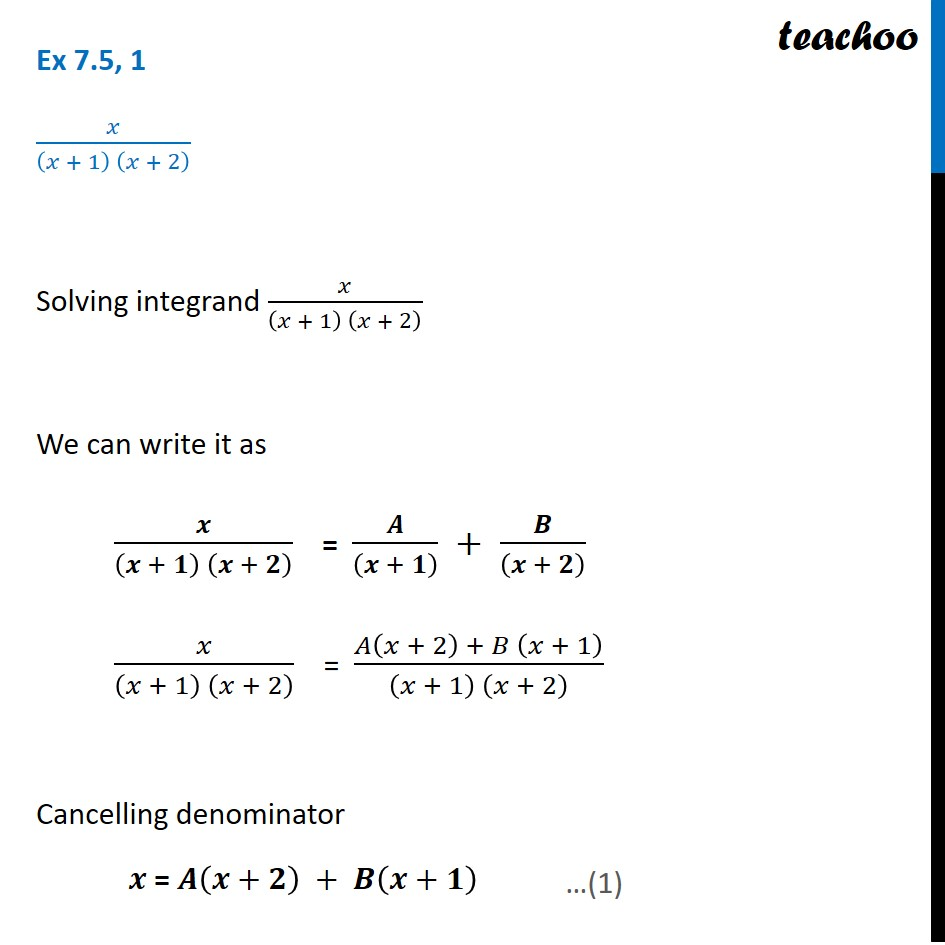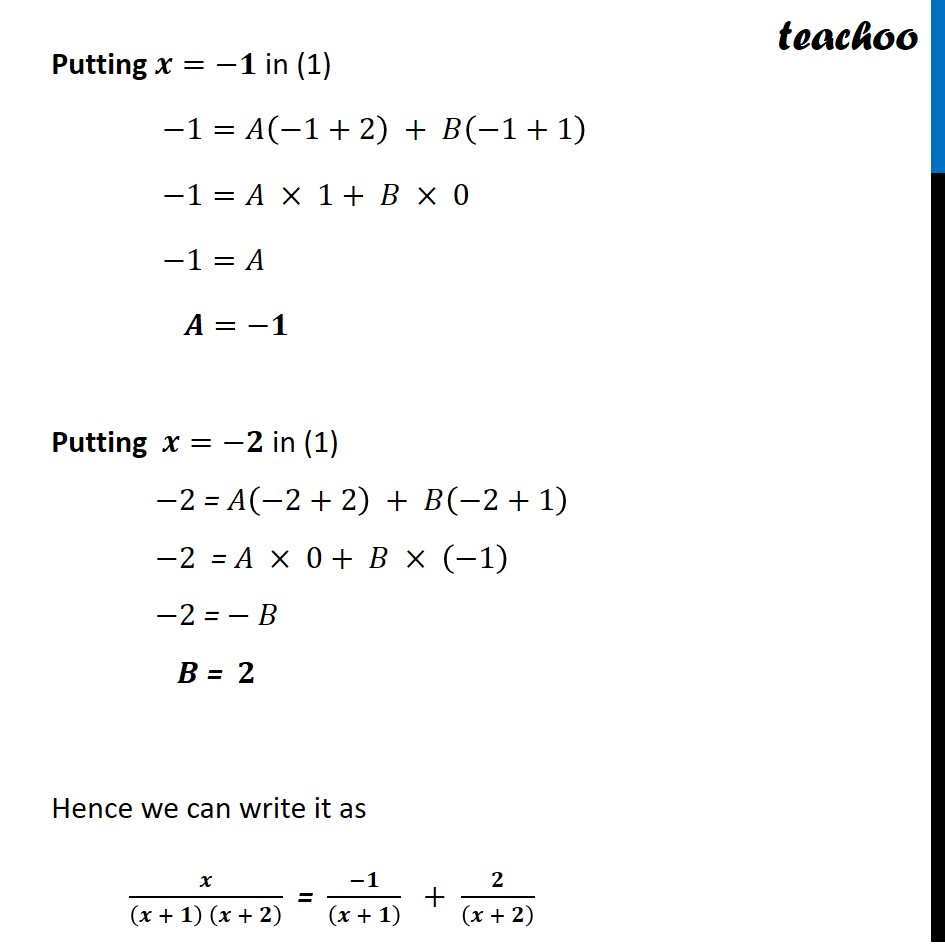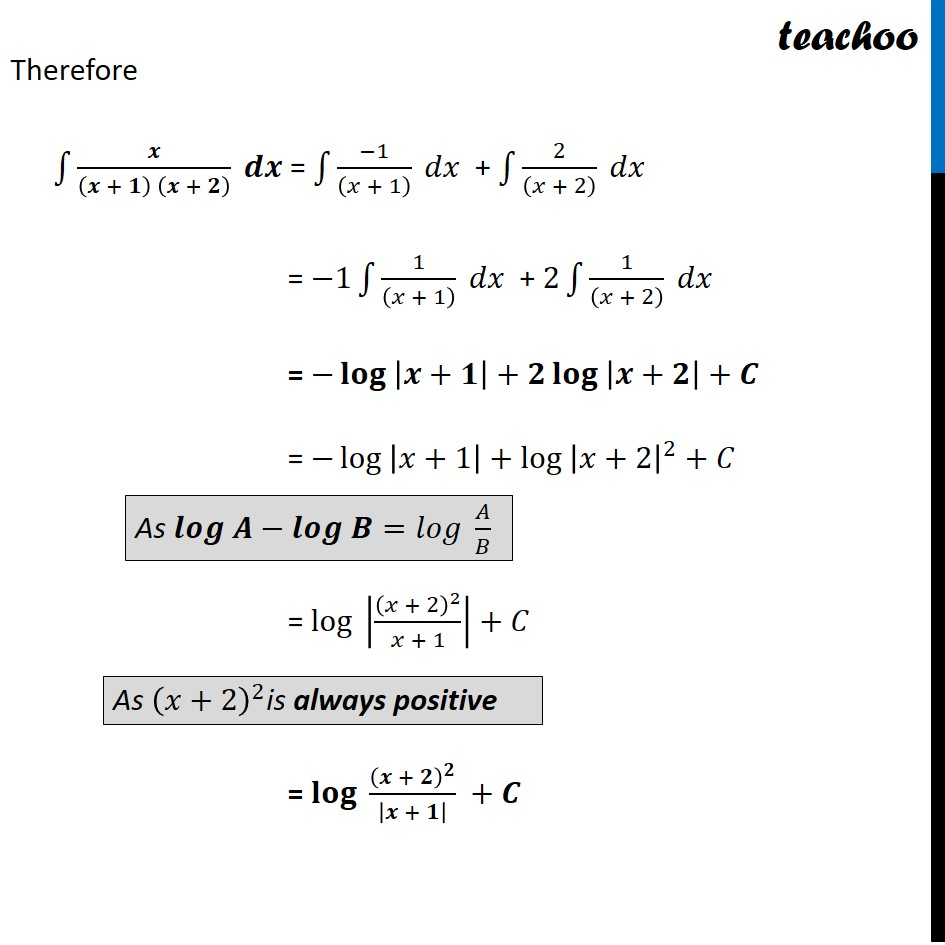Ex 7.5

Chapter 7 Class 12 Integrals
Serial order wiseLearn in your speed, with individual attention - Teachoo Maths 1-on-1 Class

### Transcript

Ex 7.5, 1 𝑥/((𝑥 + 1) (𝑥 + 2) ) Solving integrand 𝑥/((𝑥 + 1) (𝑥 + 2) ) We can write it as 𝒙/((𝒙 + 𝟏) (𝒙 + 𝟐) ) " " = 𝑨/((𝒙 + 𝟏) ) + 𝑩/((𝒙 + 𝟐) ) 𝑥/((𝑥 + 1) (𝑥 + 2) ) " " = (𝐴(𝑥 + 2) + 𝐵 (𝑥 + 1))/((𝑥 + 1) (𝑥 + 2) ) Cancelling denominator 𝒙 = 𝑨(𝒙+𝟐) + 𝑩(𝒙+𝟏) Putting 𝒙=−𝟏 in (1) −1=𝐴(−1+2) + 𝐵(−1+1) −1=𝐴 × 1+ 𝐵 × 0 −1=𝐴 𝑨=−𝟏 Putting 𝒙=−𝟐 in (1) −2 = 𝐴(−2+2) + 𝐵(−2+1) −2 = 𝐴 × 0+ 𝐵 × (−1) −2 = − 𝐵 𝑩 = 𝟐 Hence we can write it as 𝒙/((𝒙 + 𝟏) (𝒙 + 𝟐) ) = (−𝟏)/((𝒙 + 𝟏) ) + 𝟐/((𝒙 + 𝟐) ) Therefore ∫1▒𝒙/((𝒙 + 𝟏) (𝒙 + 𝟐) ) 𝒅𝒙 = ∫1▒(−1)/((𝑥 + 1) ) 𝑑𝑥 + ∫1▒2/((𝑥 + 2) ) 𝑑𝑥 = −1∫1▒1/((𝑥 + 1) ) 𝑑𝑥 + 2∫1▒1/((𝑥 + 2) ) 𝑑𝑥 = −〖𝐥𝐨𝐠 〗⁡|𝒙+𝟏|+𝟐 〖𝐥𝐨𝐠 〗⁡|𝒙+𝟐|+𝑪 = −〖log 〗⁡|𝑥+1|+〖log 〗⁡〖|𝑥+2|^2 〗+𝐶 = 〖log 〗⁡|(𝑥 + 2)^2/(𝑥 + 1)|+𝐶 = 〖𝐥𝐨𝐠 〗⁡〖(𝒙 + 𝟐)^𝟐/|𝒙 + 𝟏| 〗 +𝑪 As 𝒍𝒐𝒈 𝑨−𝒍𝒐𝒈 𝑩=𝑙𝑜𝑔 𝐴/𝐵 As (𝑥+2)^2is always positive